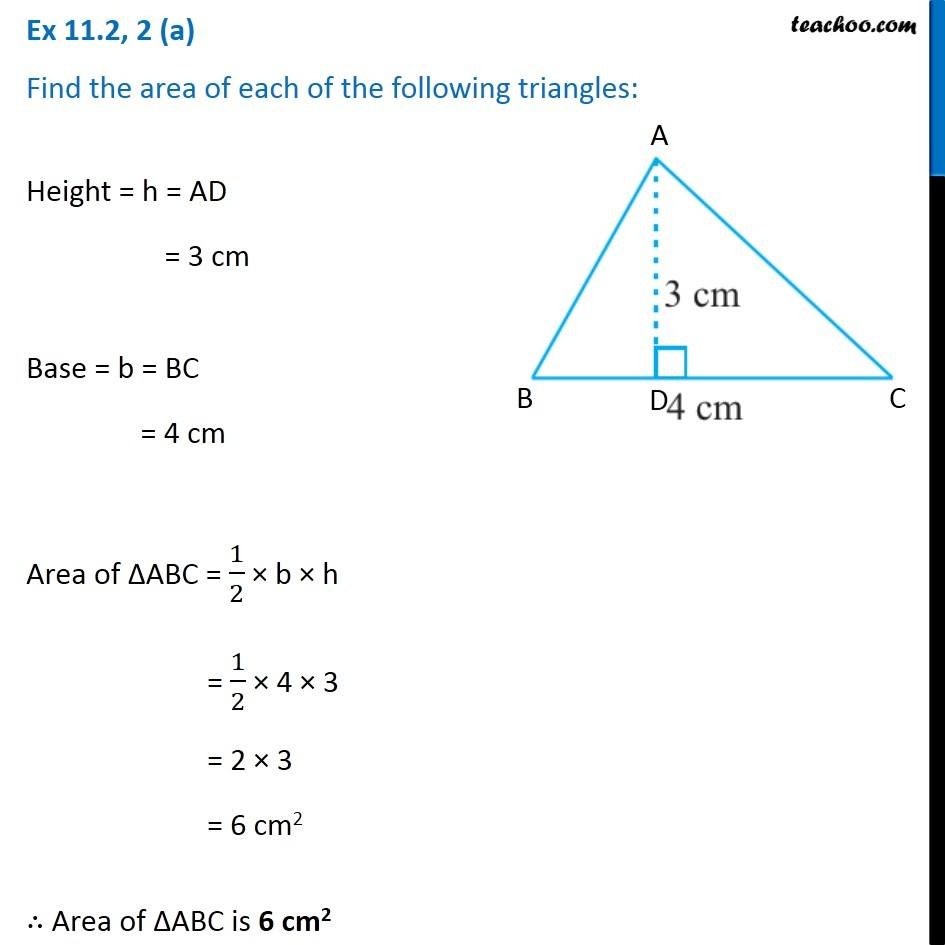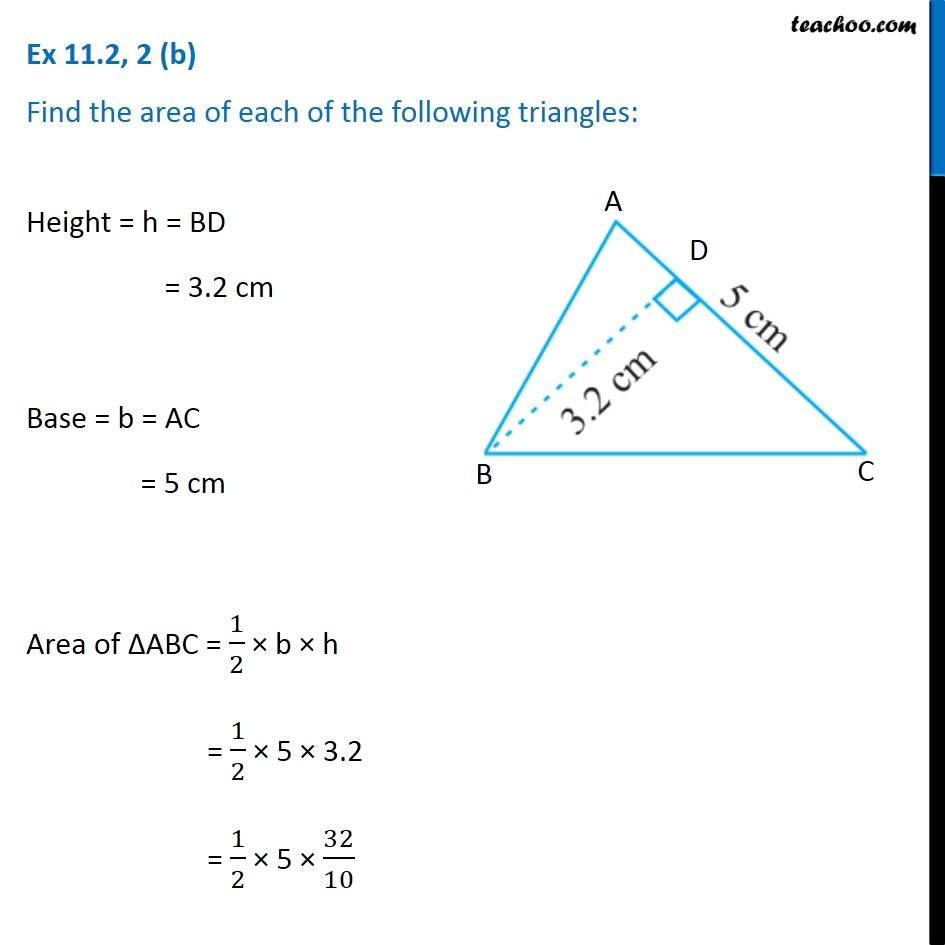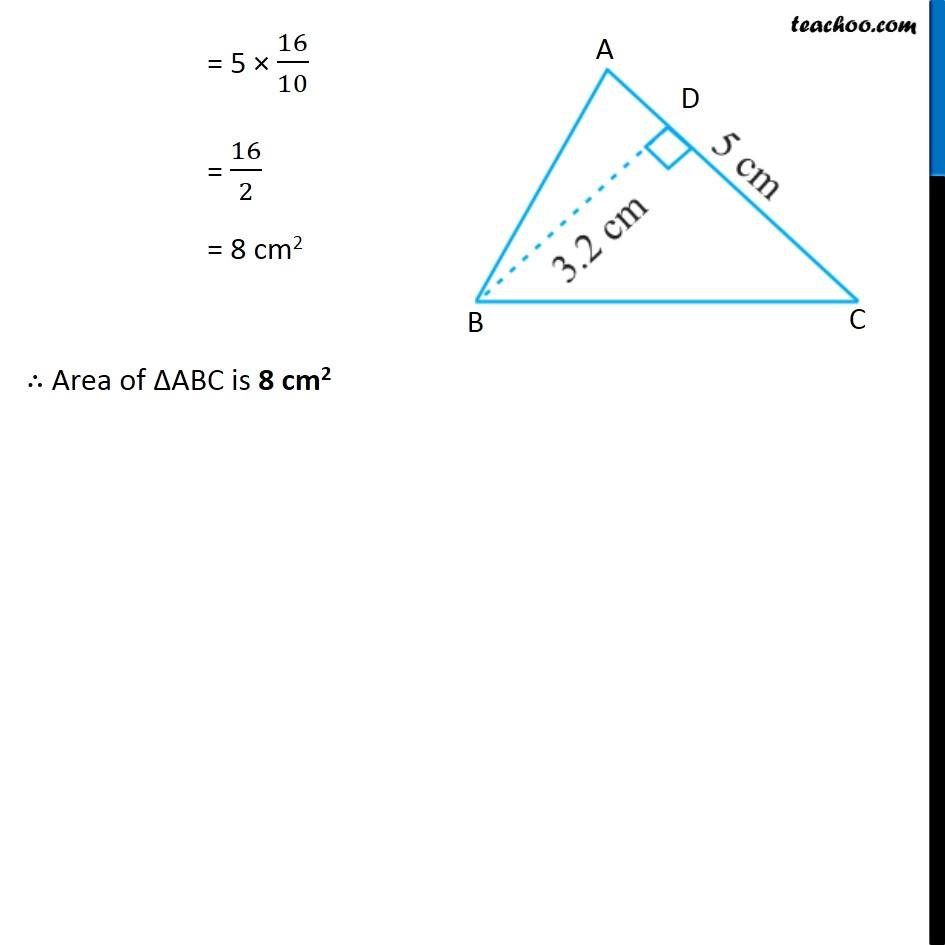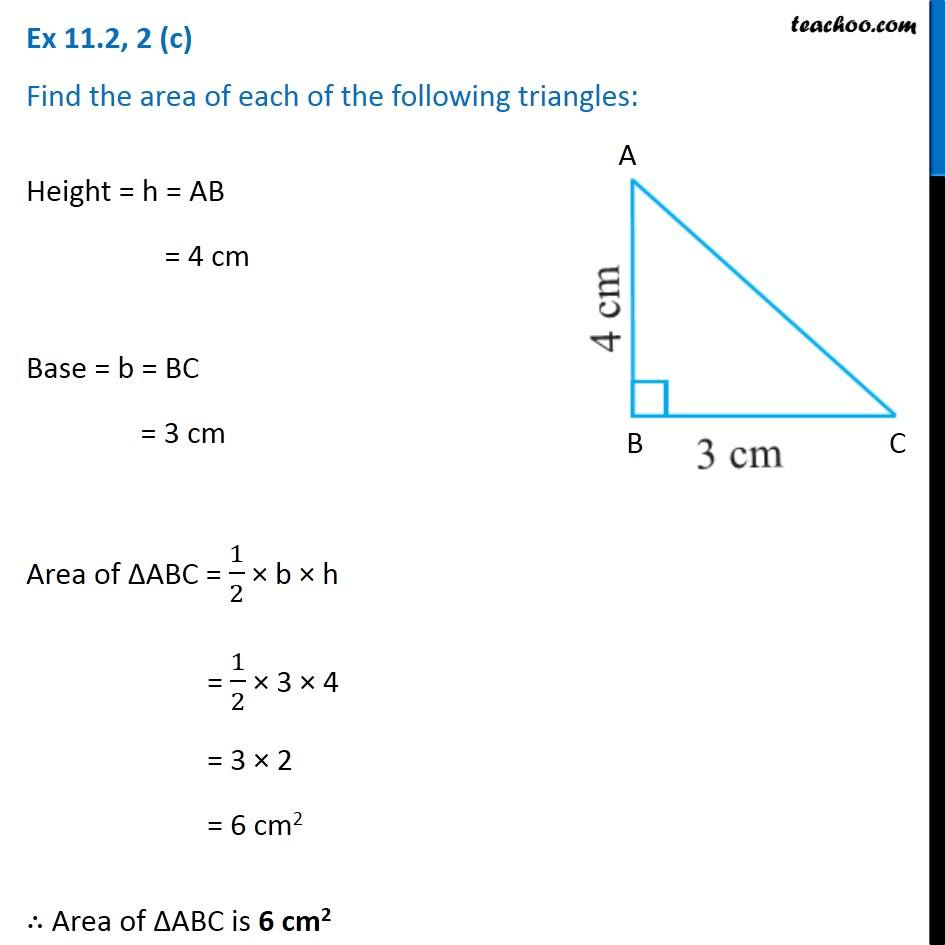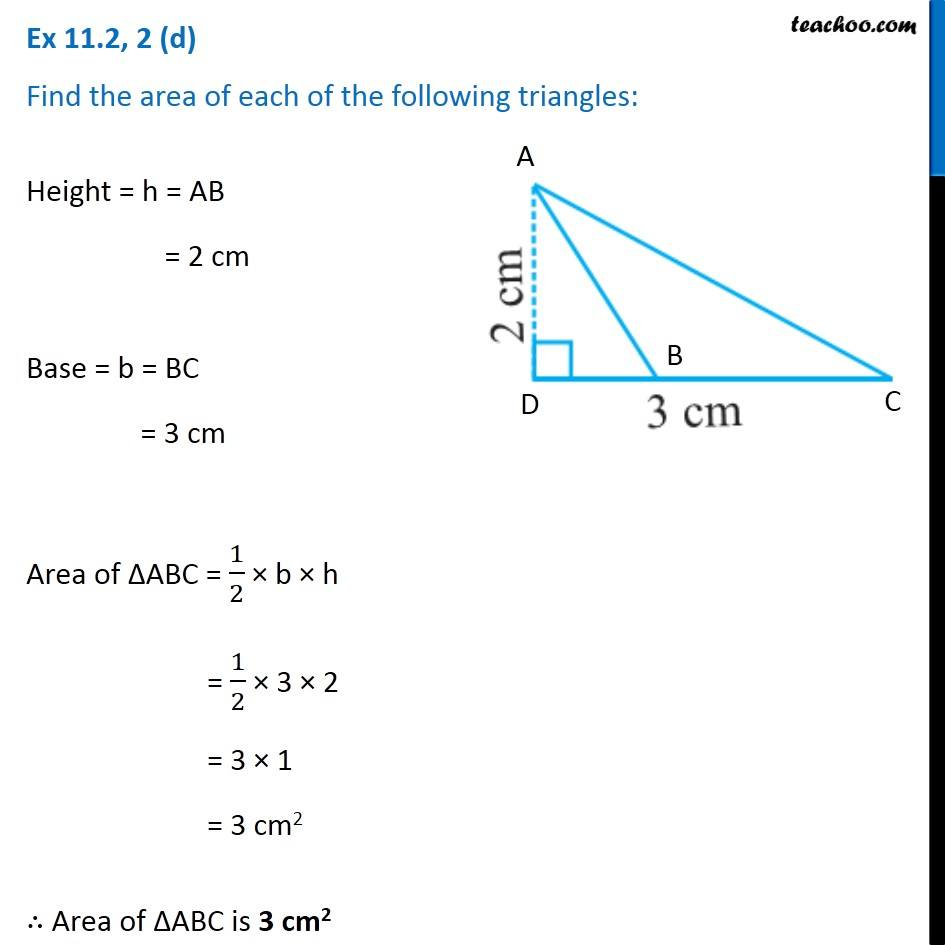Subscribe to our Youtube Channel - https://you.tube/teachoo

1. Chapter 11 Class 7 Perimeter and Area
2. Serial order wise
3. Ex 11.2

Transcript

Ex 11.2, 2 (a) Find the area of each of the following triangles: Height = h = AD = 3 cm Base = b = BC = 4 cm Area of ∆ABC = 1/2 × b × h = 1/2 × 4 × 3 = 2 × 3 = 6 cm2 ∴ Area of ∆ABC is 6 cm2 Ex 11.2, 2 (b) Find the area of each of the following triangles: Height = h = BD = 3.2 cm Base = b = AC = 5 cm Area of ∆ABC = 1/2 × b × h = 1/2 × 5 × 3.2 = 1/2 × 5 × 32/10 = 5 × 16/10 = 16/2 = 8 cm2 ∴ Area of ∆ABC is 8 cm2 Ex 11.2, 2 (b) Find the area of each of the following triangles: Height = h = BD = 3.2 cm Base = b = AC = 5 cm Area of ∆ABC = 1/2 × b × h = 1/2 × 5 × 3.2 = 1/2 × 5 × 32/10 = 5 × 16/10 = 16/2 = 8 cm2 ∴ Area of ∆ABC is 8 cm2 Ex 11.2, 2 (c) Find the area of each of the following triangles: Height = h = AB = 4 cm Base = b = BC = 3 cm Area of ∆ABC = 1/2 × b × h = 1/2 × 3 × 4 = 3 × 2 = 6 cm2 ∴ Area of ∆ABC is 6 cm2 Ex 11.2, 2 (d) Find the area of each of the following triangles: Height = h = AB = 2 cm Base = b = BC = 3 cm Area of ∆ABC = 1/2 × b × h = 1/2 × 3 × 2 = 3 × 1 = 3 cm2 ∴ Area of ∆ABC is 3 cm2

Ex 11.2